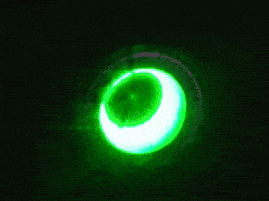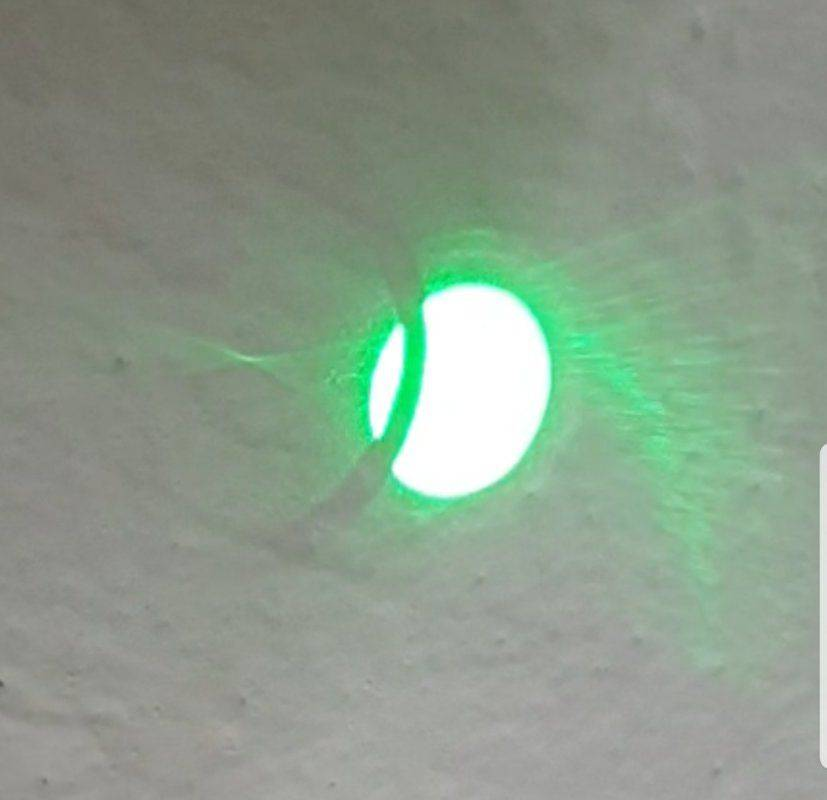Daniel Petka
TL;DR Summary
The poisson spot behaves like a miniature parabolic mirror.
Recently, I started to experiment with a laser and a coin used as a lens, being inspired by an old Cody's Lab video. My initial assumption was that through diffraction, the laser will be focused onto a spot on when the coin is a certain distance away from the wall. In a way, I imagined it as an actual lens, wrongly. The point stayed there no matter how far I moved the coin lens. Then things became even more peculiar.What is going on with the poisson spot... As you can see, it awfully resembles a parabolic mirror. This becomes more apparent when you use a ring instead of the coin.If you have any ideas, I'd love to be hearing from you. Cheers.

#### Attachments

Gold Member
I'm trying to make some sense of this. How can a coin resemble a lens? Also, what is it that looks like a "parabolic mirror"? A diagram might have helped. Remember, PF is not psychic and we can only react to what is posted. What's actually going on in those images?

The Poisson Spot is what can appear in the shadow of a small opaque sphere. There is no magic involved, once you understand how the Maths of Refraction works. What have you read about this so far? We need to know if you are going to get an appropriate answer.

Cutter Ketch
Let me see if I understand your question. You are looking at the diffraction from the edge of a coin inserted into a wide top hat profile laser beam. Because the edge is curved, the edge diffraction is converging, and on a screen you get a cone of light converging to a tight spot. In analogy to a lens you expected that the tight spot would only occur when the coin was a certain distance from the screen, but you found, to your surprise, that the diffraction converged to a tight spot no matter what distance you placed the coin from the screen and you want to know why. Correct?

If I have that right, here is why. Suppose the light diffracted around the edge only at one precise angle. The curved edge would then produce a precise cone of light which would converge to a tight spot at exactly one distance behind the coin. This would be a focus like you expected. The focal length is determined by the curvature of the edge and the angle of diffraction.

Now, in actuality the light diffracts around the edge into a whole range of angles, more light at smaller angles, less at larger angles, but a whole spray. For each angle there is a focal length. If you put the screen at a large distance then the bright diffraction of the shallow angles are focused and the spot is closer to the edge (in angle space). The larger angles deeper into the shadow are further and further past focus. You get a triangle of light diverging deeper into the shadow. At a shorter distance, larger diffraction angles are focused. The shallower angles closer to the edge of the shadow are more and more inside their focus. You get a triangle of light diverging as you get closer to the edge.

Because the diffraction is brighter at shallower angles the triangle towards the edge is more prominent than the triangle deeper into the shadow.

As you move the coin closer and further from the screen you should see the spot move further and closer to the edge of the shadow.

Gold Member
The curved edge would then produce a precise cone of light which would converge to a tight spot at exactly one distance behind the coin.
I see where you're going with this. But, unlike a spherical lens in which can be a high level of what is referred to as spherical distortion, for a solid disc or sphere there is a massive range of distances where you get some form of 'focus'. Right in close there is a shadow and at a great distance there is no peak (afaik). The Fraunhoffer (distant) pattern of a round aperture is an Airey Disc and the inverse pattern for a solid disc will have a hole of the same angular size in the centre. The Fresnel pattern seems to be what you get in the near field and has the (fuzzy) Poisson spot.
The diffraction pattern of a neutral density sphere (no change of refractive index) would presumably resemble the inverse of the pattern of an opaque sphere at infinity.

Cutter Ketch
for a solid disc or sphere there is a massive range of distances where you get some form of 'focus'.

Did you even read the post? The hypothetical is correct and matches what he thought should happen. The extrapolation to the real situation is also correct. And guess what, you get a massive range of distances where you get some sort of focus.